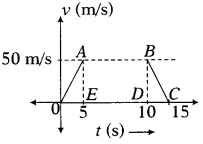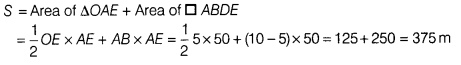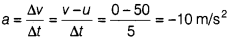# The distance travelled by the object in 10 s

Following graph represents the velocity-time graph of an object of mass 20 kg.Find out

1. the distance travelled by the object in 10 s.
2. the force on object from t = 10stot = 15s.
1. Since, we know that distance covered by a body,
Distance = Area under velocity - time graph
∴ The distance covered by the object from t = 0 to t = 10 s.2. From the graph, the object comes to rest at t =15s.
Speed of the object at t = 10 s, u = 50 m/s
Speed of the object at t = 15 s, v = 0
and the time interval for change in speed, Δt = 15 - 10 = 5s
∴ Acceleration of the object,Since, force, F = mass x acceleration = 20 x -10 = -200 N
The negative sign implies that the force is in opposite direction to the velocity of the objecet.